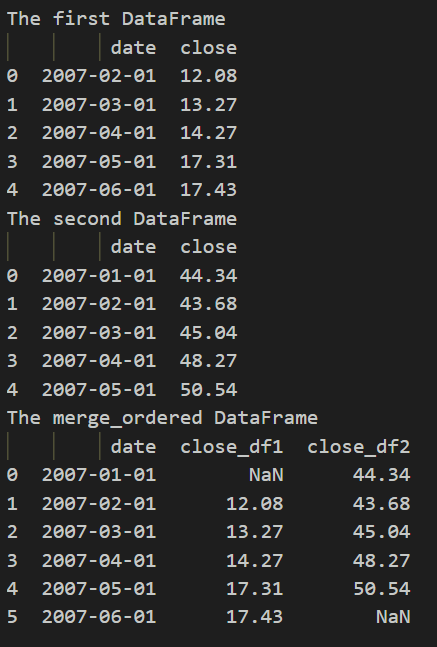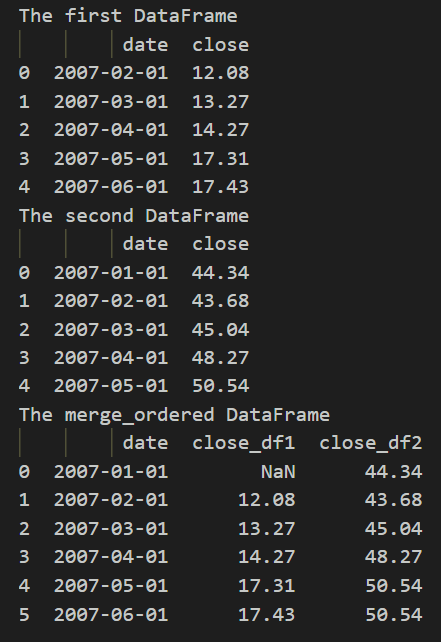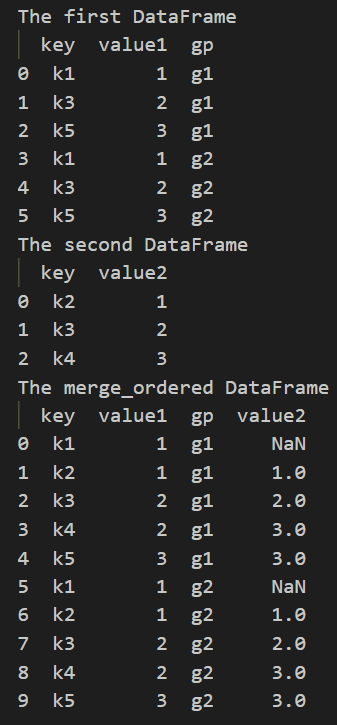Python | pandas.merge_ordered() function

• Last Updated : 22 Sep, 2021

This method is used to designed for ordered data like time series data. Optionally perform group-wise merge.

Syntax : pandas.merge_ordered(left, right, on=None, left_on=None, right_on=None, left_by=None, right_by=None, fill_method=None, suffixes=(‘_x’, ‘_y’), how=’outer’)
Parameters :

• left : DataFrame
• right : DataFrame
• on : label or list
• left_on : label or list, or array-like
• right_on : label or list, or array-like
• left_by : column name or list of column names
• right_by : column name or list of column names
• fill_method : {‘ffill’, None}, default None
• suffixes : list-like, default is (“_x”, “_y”)
• how : {‘left’, ‘right’, ‘outer’, ‘inner’}, default ‘outer’

Returns : A DataFrame in which the merged DataFrame output type will the be same as ‘left’, if it is a subclass of DataFrame.

Example 1 : merge ordered two Dataframe with same number of elements

Python3

 # importing the moduleimport pandas as pd  # creating the first DataFramedf1 = pd.DataFrame({    "date": ['2007-02-01', '2007-03-01', '2007-04-01', '2007-05-01', '2007-06-01'],    "close": [12.08, 13.27, 14.27, 17.31, 17.43]})print("The first DataFrame")print(df1)  # creating the second DataFramedf2 = pd.DataFrame({    "date": ['2007-01-01', '2007-02-01', '2007-03-01', '2007-04-01', '2007-05-01'],    "close": [44.34, 43.68, 45.04, 48.27, 50.54]})print("The second DataFrame")print(df2)  # merging the DataFramesprint("The merge_ordered DataFrame")  df = pd.merge_ordered(df1, df2, on='date', suffixes=('_df1', '_df2'))  print(df)# This code is contributed by rakeshsahni

Output :Example 2 : fills missing with previous value we use fill_method = ‘ffill’ ( Forward fill )

Python3

 # importing the moduleimport pandas as pd  # creating the first DataFramedf1 = pd.DataFrame({    "date": ['2007-02-01', '2007-03-01', '2007-04-01', '2007-05-01', '2007-06-01'],    "close": [12.08, 13.27, 14.27, 17.31, 17.43]})print("The first DataFrame")print(df1)  # creating the second DataFramedf2 = pd.DataFrame({    "date": ['2007-01-01', '2007-02-01', '2007-03-01', '2007-04-01', '2007-05-01'],    "close": [44.34, 43.68, 45.04, 48.27, 50.54]})print("The second DataFrame")print(df2)  # merging the DataFramesprint("The merge_ordered DataFrame")  df = pd.merge_ordered(df1, df2, on='date', suffixes=(    '_df1', '_df2'), fill_method='ffill')  print(df)# This code is contributed by rakeshsahni

Output :Example 3 : we will use left_by parameter in which group left DataFrame by group columns and merge piece by piece with right DataFrame.

Python3

 # importing the moduleimport pandas as pd  # creating the first DataFramedf1 = pd.DataFrame(    {        "key": ["k1", "k3", "k5", "k1", "k3", "k5"],        "value1": [1, 2, 3, 1, 2, 3],        "gp": ["g1", "g1", "g1", "g2", "g2", "g2"]    })df1print("The first DataFrame")print(df1)  # creating the second DataFramedf2 = pd.DataFrame({"key": ["k2", "k3", "k4"], "value2": [1, 2, 3]})  print("The second DataFrame")print(df2)  # merging the DataFramesprint("The merge_ordered DataFrame")  df = pd.merge_ordered(df1,df2,fill_method='ffill',left_by="gp")  print(df)# This code is contributed by rakeshsahni

Output :Attention geek! Strengthen your foundations with the Python Programming Foundation Course and learn the basics.

To begin with, your interview preparations Enhance your Data Structures concepts with the Python DS Course. And to begin with your Machine Learning Journey, join the Machine Learning - Basic Level Course

My Personal Notes arrow_drop_up